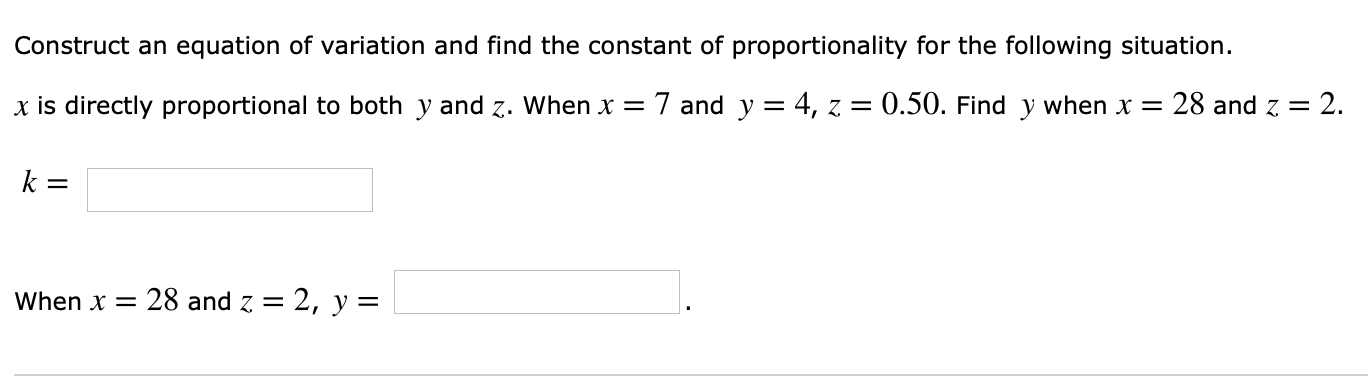Construct an equation of variation and find the constant of proportionality for the following situation.x is directly proportional to both y and z. when x7 and y = 4, z = 0.50. Find y when x = 28 and Z = 2.when x = 28 and Z = 2, y =

Questionhelp_outlineImage TranscriptioncloseConstruct an equation of variation and find the constant of proportionality for the following situation. x is directly proportional to both y and z. when x 7 and y = 4, z = 0.50. Find y when x = 28 and Z = 2. when x = 28 and Z = 2, y = fullscreen
Step 1

It is given that, x is directly proportional to y and z.

Step 2

Now substitute x = 7, y = 4 and z = 0.5 and compute the constant k as follows.

Step 3

Substitute k = 7/2 in the equa...

Want to see the full answer?

See Solution

Want to see this answer and more?

Our solutions are written by experts, many with advanced degrees, and available 24/7

See Solution
Tagged in

Algebra